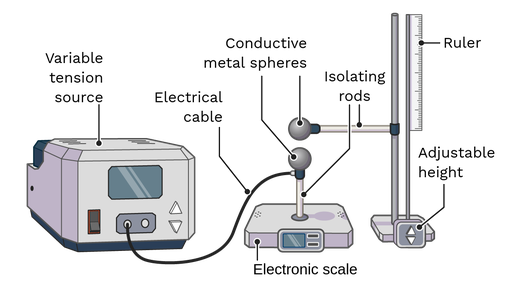Coulomb’s experiment is designed to measure the electrostatic force between two charged spheres. The experiment was first performed by Charles-Augustin de Coulomb in 1785 using a torsion balance. Since then, there have been multiple visions of the experiment. Here we present a simplified version that doesn’t require prior knowledge of trigonometry.

The experimental setup consists of:

• A digital scale
• Two conductive spheres, each connected to an insulating rod from which it can be handled without risk of discharge
• A measuring rod with support for one of the spheres
• A method for transferring charge to the spheres

The experiment follows these steps:

1. Place one of the spheres on the digital scale (on an isolating platform if needed).
2. Set the tare of the scale to the weight of the sphere and its insulation.
3. Set the scale to give its measurement in Newtons.
4. Using the support on the measuring rod, place the second sphere at a distance above the first, on the same vertical line.
5. Give the spheres a charge using your preferred charging method, for example, connect them to a tension source or a charged object.

The reading on the scale will measure the electrostatic force and vary with the charges of the spheres and the distance between their centers as established by Coulomb’s law. The reading on the scale will be the magnitude of the electrostatic force, with a minus sign if the spheres attract and a plus sign if they repel.Figure: Possible setup for the variation of Coulomb's experiment described above.